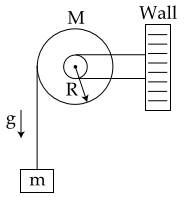#### A uniform disc of radius R and mass M is free to rotate only about its axis.  A string is wrapped over its rim and a body of mass m is tied to the free end of the string as shown in the figure.  The body is released from rest.  Then the acceleration of the body is :Option 1)Option 2)Option 3)Option 4)As we learnt in

Analogue of second law of motion for pure rotation -- wherein

Torque equation can be applied only about two points

(i) centre of motion.

(ii) point which has zero velocity/acceleration.

Short trickOption 1)This is the correct oiption.

Option 2)This is an incorrect option.

Option 3)This is an incorrect option.

Option 4)This is an incorrect option.

#### perimeter